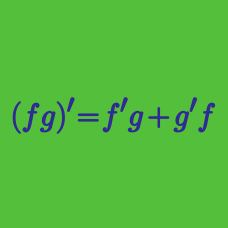Calculus

# Differentiation - Quotient Rule

If $$f(x) = \frac{2x + 4}{3x + 7}$$, what is the value of $$f'(3)$$?

If $$f(x)=\frac{-9x}{x^2+5}$$, what is the value of $$f'(5)$$?

Let $$g(x)$$ be a differentiable function such that $$g(0)=2.$$ If $$\displaystyle f(x)=\frac{1}{xg(x)+6},$$ where what is the value of $$f'(0)?$$

Let $$g(x)$$ be a differentiable function such that $$g(x) \neq -7.$$ If $$f(x)=\frac{x}{7+g(x)}$$ and $$f'(0)=\frac{1}{14},$$ what is the value of $$g(0)?$$

If $$a$$ and $$b$$ are constants such that $$f(x)=\frac{ax+b}{x^2+x+1}$$ and $f'(0)=-3, \; f'(-1)=10,$ what is $$2a+b?$$

×